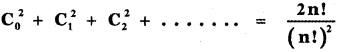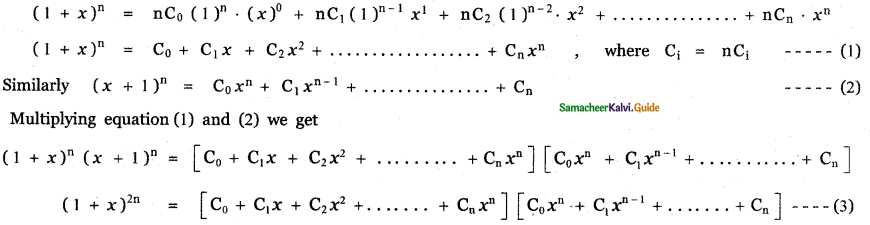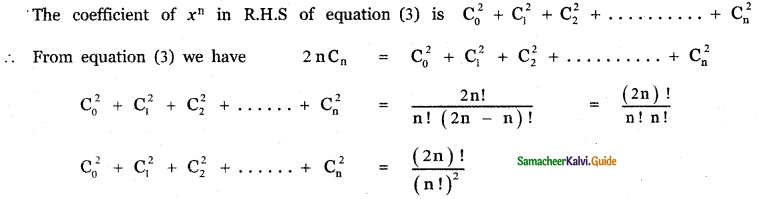Tamilnadu State Board New Syllabus Samacheer Kalvi 11th Maths Guide Pdf Chapter 5 Binomial Theorem, Sequences and Series Ex 5.1 Text Book Back Questions and Answers, Notes.

## Tamilnadu Samacheer Kalvi 11th Maths Solutions Chapter 5 Binomial Theorem, Sequences and Series Ex 5.1

Question 1.
Expand
(i) $$\left(2 x^{2}-\frac{3}{x}\right)^{3}$$
(ii) $$\left(2 x^{2}-3 \sqrt{1-x^{2}}\right)^{4}+\left(2 x^{2}+3 \sqrt{1-x^{2}}\right)^{4}$$
(i) $$\left(2 x^{2}-\frac{3}{x}\right)^{3}$$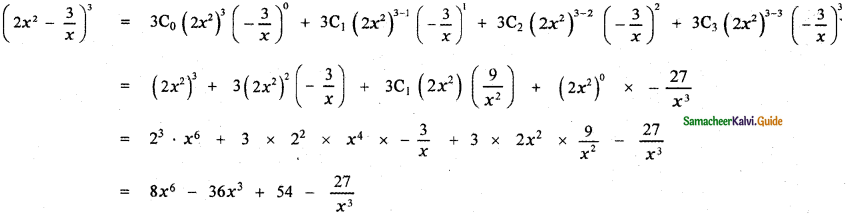(ii) $$\left(2 x^{2}-3 \sqrt{1-x^{2}}\right)^{4}+\left(2 x^{2}+3 \sqrt{1-x^{2}}\right)^{4}$$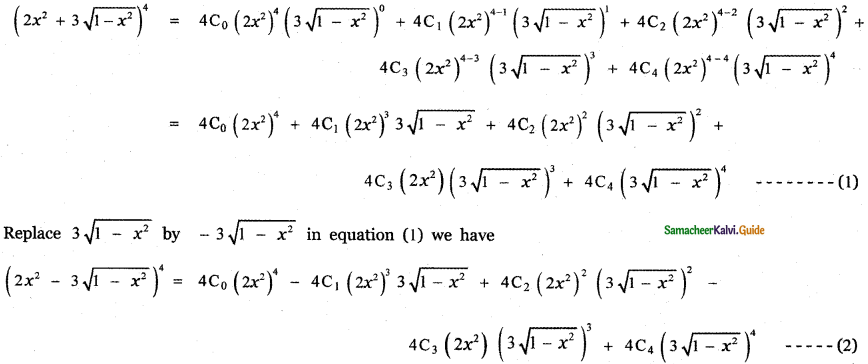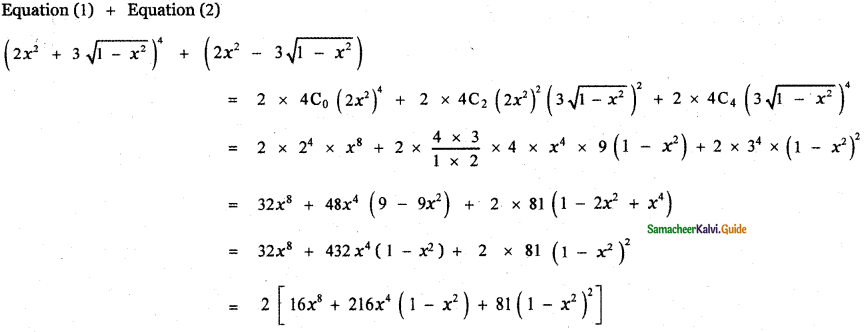Question 2.
Compute
(i) 1024
(ii) 994
(iii) 97
(i) 1024
1024 = (100 + 2)4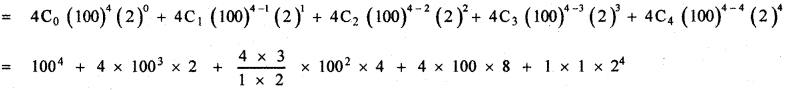= 100000000 + 8 × 1000000 + 24 × 10000 + 3200 + 16
= 100000000 + 8000000 + 240000 + 3216
= 108243216(ii) 994
994 = (100 – 1)4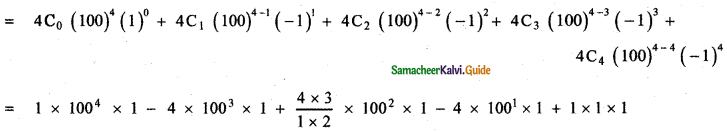= 100000000 – 4 × 1000000 + 6 × 10000 – 400 + 1
= 100000000 – 4000000 + 60000 -400+1
= 96059601

(iii) 97
97 = (10 – 1)7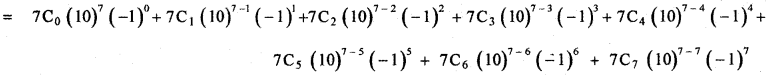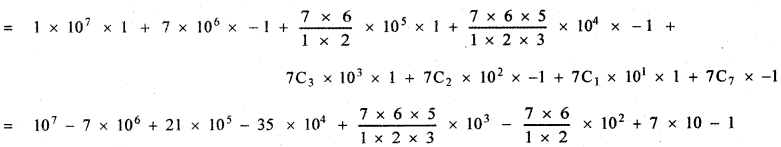= 103 (104 – 7 × 103 + 21 × 102 – 35 × 10 + 35) – 21 × 100 + 70 – 1
= 103 (10000 – 7000 + 2100 – 350 + 35 ) – 2100 + 70 – 1
= 103 (12135 – 7350) – 2031
= 103 × 4785 – 2031
= 4785000 – 2031
= 4782969

Question 3.
Using binomial theorem, indicate which of the following two number is larger. (l.Ol)1000000, 10000 Answer: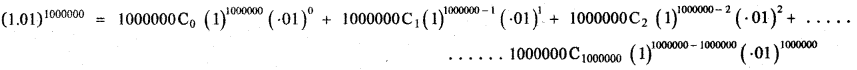= 1000000C0 + 1000000C1( . 01) + …………………….
= 1 + 1000000 × 0 . 01 + ……………………..
= 1 + 10000 + other positive terms
= 10001 + other positive terms
(1.01)1000000 – 10000 = 10001 + other positive terms – 10000 > 0
∴ (1.01)1000000 > 10000 ⇒ (1.01)1000000 is larger.Question 4.
Find the coefficient of x15 in $$\left(x^{2}+\frac{1}{x^{3}}\right)^{10}$$
General term Tr+1 = nCr xn-r . ar
∴ In the expansion $$\left(x^{2}+\frac{1}{x^{3}}\right)^{10}$$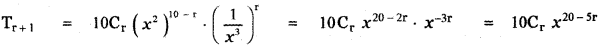To find the coefficient of x15, Put 20 – 5r = 15
20 – 15 = 5r ⇒ 5r = 5 ⇒ r = 1
∴ T1 + 1 = 10C1x20 – 5 ⇒ T2 = 10 . x15
∴ The coefficient of x15 is 10

Question 5.
Find the coefficient of x6 and the coefficient of x2 in $$\left(x^{2}-\frac{1}{x^{3}}\right)^{6}$$
The general terms is Tr+1 = nCran-r . ar
∴ The general term in the expansion of $$\left(x^{2}-\frac{1}{x^{3}}\right)^{6}$$ is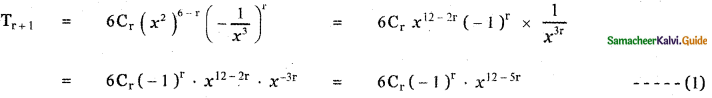To find the coefficient of x6 , Put 12 – 5r = 6
12 – 6 = 5r ⇒ 5r = 6 ⇒ r = $$\frac{6}{5}$$ which is impossible.
∴ There is no x6 term in the expansion.
To find the coefficient of x2,
Put 12 – 5r = 2
⇒ 12 – 2 = 5r
⇒ 10 = 5r
⇒ r = $$\frac{10}{5}$$ = 5
Substituting in (1) we have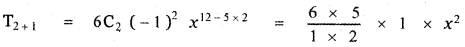T3 = 15
∴ The coefficient of x2 is 15Question 6.
Find the coefficient of x4 in the expansion of (1 + x3)50 $$\left(x^{2}+\frac{1}{x}\right)^{5}$$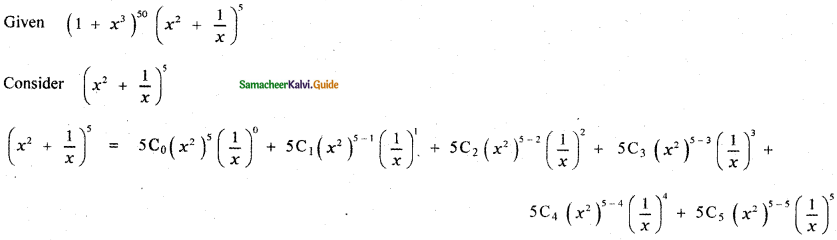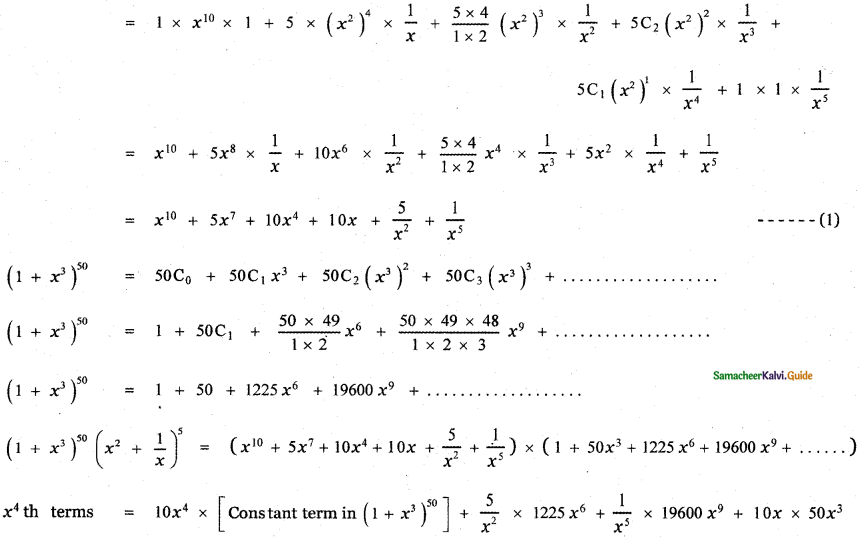= 10x4 × 1 + 5 × 1225 x4 + 19600 x4 + 500 x4
= x4 (10 + 6125 + 19600 + 500) = 26235 . x4
∴ The coefficient of x4 is 26235.Question 7.
Find the constant term of $$\left(x^{3}-\frac{1}{3 x^{2}}\right)^{5}$$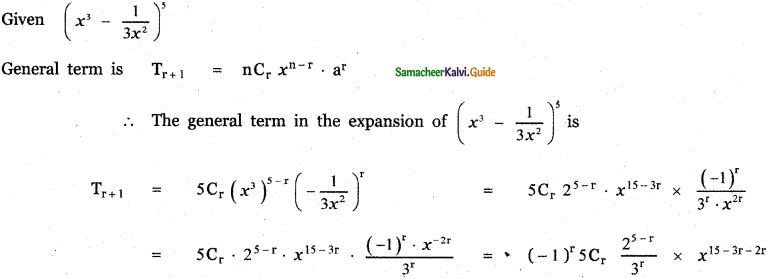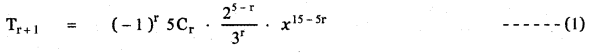To get the constant term,
Put 15 – 5r = 0
⇒ 5r = 15
⇒ r = 3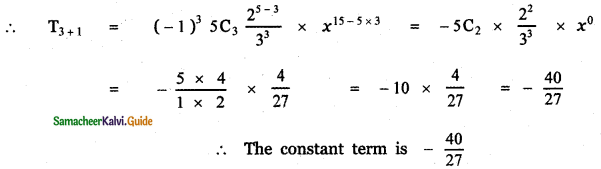Question 8.
Find the last two digits of the number 3600.
Consider 3600
3600 = (32)300 = 9300 = (1o – 1)300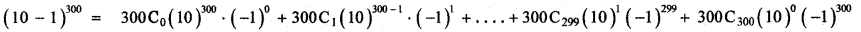= 10300 – 300 (10)299 + ………………. + 300 C1 × 10 × – 1 + 1 × 1 × 1
= 10300 – 300 (1o)299 + …………….. – 300 × 10 + 1
= 10300 – 300 × 10299 + ……………… – 3000 + 1
All the terms except the last are multiples of 100 and hence divisible by 100.
∴ The last two digits will be 01.

Question 9.
If n is a positive integer show that 9n+1 – 8n – 9 is always divisible by 64.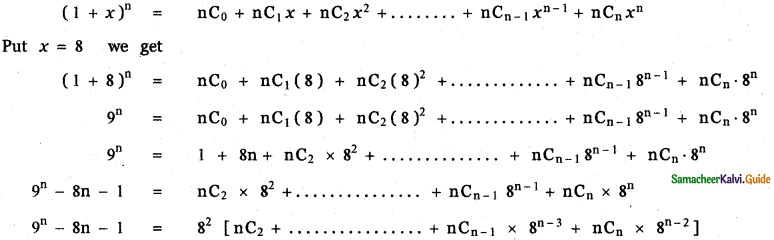which is divisible by 64 for all positive integer n.
∴ 9n – 8n – 1 is divisible by 64 for all positive integer n.
Put n = n + 1 we get
9n + 1 – 8 (n + 1) – 1 is divisible by 64 for all possible integer n
(9n + 1 – 8n – 8 – 1) is divisible by 64
∴ 9n + 1 – 8n – 9 is always divisible by 64Question 10.
If n is an odd positive integer, prove that the coefficients of the middle terms in the expansion of (x + y)n are equal.
Given (x + y)n
If n is odd the middle term in the expansion of (x + y)n are $$\frac{T_{n-1}}{2}+1$$ and $$\mathrm{T}_{\frac{\mathrm{n}+1}{2}+1}$$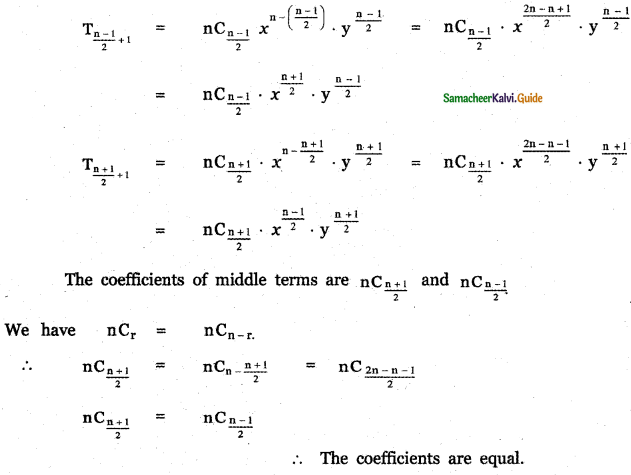Question 11.
If n is a positive integer and r is a non-negative integer, prove that the coefficients of xr and xn-r in the expansion of (1 + x)n are equal.
Given (1 + x)n.
General term Tr+1 = nCr xn – r . ar
∴ The general term in the expansion of (1 + x)n is Tr+1 = nCr . (1)n – r . xr
Tr+1 = nCr . xr ……….. (1)
∴ Coefficient of xr is nCr,
Put r = n – r in (1)
Tn – r + 1 = nCn – r . xn-r
∴ The coefficient of xn-r is nCn-r …………. (2)
we know nCr = nCn-r
∴ The coefficient of xr and coefficient of xn-r are equal, (by (1) & (2))Question 12.
If a and b are distinct integers, prove that a – b is a factor of an – bn, whenever n is a positive integer.
Let a = a + b – b
= b + (a – b)
an = [b + (a – b)]n
Using binomial expansion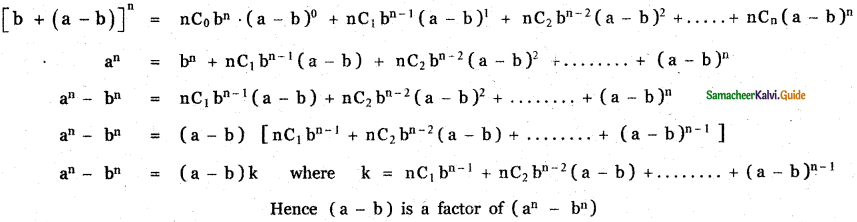Question 13.
In the binomial expansion of (a + b )n , the coefficients of the 4th and 13th terms are equal to each other , find n.
In (a + b)n general term is tr + 1 = nCr an – r br
So, t4 = t3 + 1 = nC3 = nC12
⇒ n = 12 + 3 = 15
We are given that their coefficients are equal ⇒ nC3 = nC12 ⇒ n = 12 + 3 = 15
[nCx = nCy ⇒ x = y (or) x + y = n]Question 14.
If the binomial co – efficients of three consecutive terms in the expansion of (a + x)n are in the ratio 1 : 7 : 42, then find n.
The general term in the expansion of (a + b)n is Tr+1 = nCr . an-r . br
∴ The general term in the expansion of (a + x)n is Tr+1 = nCr . an-r . xr
Let the three consecutive terms be rth term, (r + 1 )th term, (r + 2 )th term.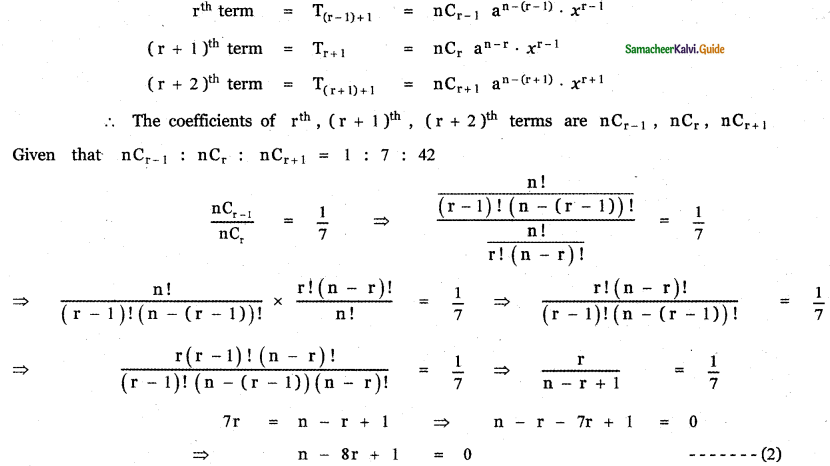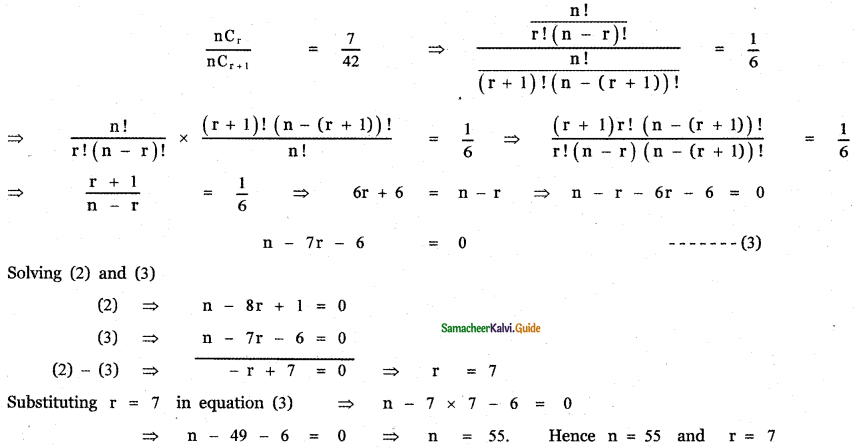Question 15.
In the binomial coefficients of (1 + x)n, the coefficients of the 5th, 6th, and 7th terms are in A.P. Find all values of n.
Given (1 + x)n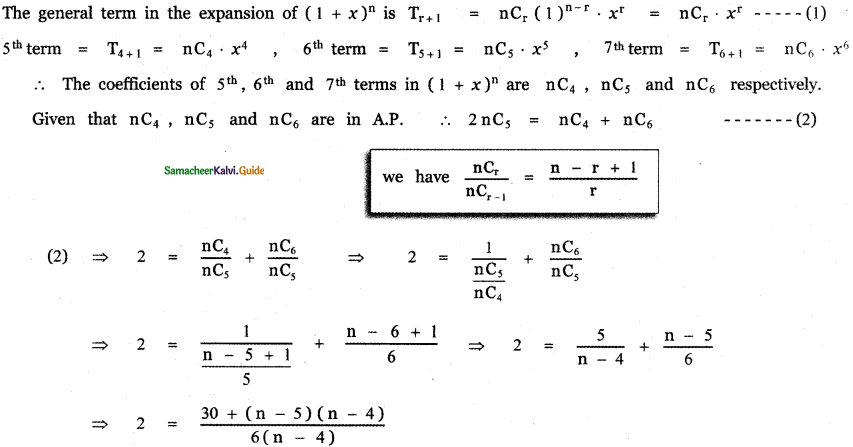12(n – 4) = 30 + n2 – 5n – 4n + 20 ⇒ 12n – 48 = 30 + n2 – 9n + 20
⇒ n2 – 9n – 12n + 50 + 48 = 0 ⇒ n2 – 21n + 98 = 0
⇒ n2 – 14n – 7n + 98 = 0 ⇒ n(n – 14) – 7(n – 14) = 0
⇒ (n – 7) (n – 14) = 0 ⇒ n = 7 or n = 14

Question 16.
prove that